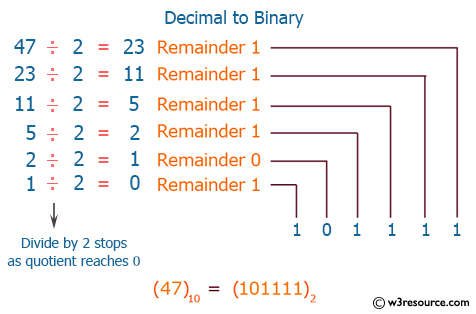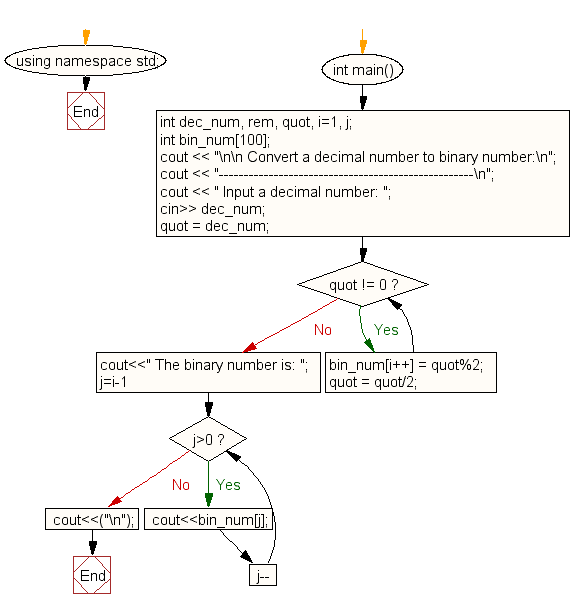﻿ C++ Exercises: Convert a decimal number to binary number - w3resource# C++ Exercises: Convert a decimal number to binary number

## C++ For Loop: Exercise-70 with Solution

Write a program in C++ to convert a decimal number to binary number.

Pictorial Presentation:Sample Solution:-

C++ Code :

``````#include <iostream>
#include <math.h>
using namespace std;

int main()
{
int dec_num, rem, quot, i=1, j;
int bin_num;

cout << "\n\n Convert a decimal number to binary number:\n";
cout << "---------------------------------------------------\n";
cout << " Input a decimal number: ";
cin>> dec_num;
quot = dec_num;
while(quot != 0)
{
bin_num[i++] = quot%2;
quot = quot/2;
}
cout<<" The binary number is: ";
for(j=i-1; j>0; j--)
{
cout<<bin_num[j];
}
cout<<("\n");
}
``````

Sample Output:

``` Convert a decimal number to binary number:
---------------------------------------------------
Input a decimal number: 35
The binary number is: 100011
```

Flowchart:C++ Code Editor:

Contribute your code and comments through Disqus.

What is the difficulty level of this exercise?

﻿